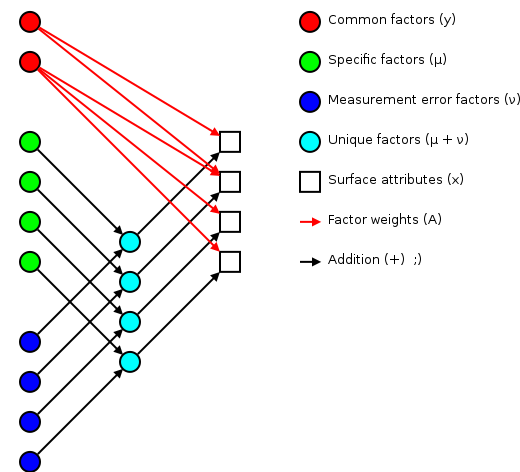I've been trying to wrap my head around factor analysis as a theory for designing and understanding test and survey results. This has turned out to be another one of those fields where the going has been a bit rough. I think the key factors in making these older topics difficult are:

• “Everybody knows this, so we don't need to write up the details.”
• “Hey, I can do better than Bob if I just tweak this knob…”
• “I'll just publish this seminal paper behind a paywall…”

The resulting discussion ends up being overly complicated, and it's hard for newcomers to decide if people using similar terminology are in fact talking about the same thing.

Some of the better open sources for background has been Tucker and MacCallum's “Exploratory Factor Analysis” manuscript and Max Welling's notes. I'll use Welling's terminology for this discussion.

The basic idea of factor analsys is to model $d$ measurable attributes as generated by $k common factors and $d$ unique factors. With $n=4$ and $k=2$, you get something like:Corresponding to the equation (Welling's eq. 1):

(1)$x=Ay+\mu +\nu$

The independent random variables $y$ are distributed according to a Gaussian with zero mean and unit variance ${𝒢}_{y}\left[0,I\right]$ (zero mean because constant offsets are handled by $\mu$; unit variance because scaling is handled by $A$). The independent random variables $\nu$ are distributed according to ${𝒢}_{\nu }\left[0,\Sigma \right]$, with (Welling's eq. 2):

(2)$\Sigma \equiv \text{diag}\left[{\sigma }_{1}^{2},\dots ,{\sigma }_{d}^{2}\right]$

The matrix $A$ (linking common factors with measured attributes $x\right)\mathrm{is}\mathrm{refered}\mathrm{to}\mathrm{as}\mathrm{the}\mathrm{factor}\mathrm{weights}\mathrm{or}\mathrm{factor}\mathrm{loadings}.\mathrm{Because}\mathrm{the}\mathrm{only}\mathrm{source}\mathrm{of}\mathrm{constant}\mathrm{offset}\mathrm{is}$\mathbf{\mu}$,\mathrm{we}\mathrm{can}\mathrm{calculate}\mathrm{it}\mathrm{by}\mathrm{averaging}\mathrm{out}\mathrm{the}\mathrm{random}\mathrm{noise}\left(\mathrm{Welling}\prime s\mathrm{eq}.6\right):\text{Unknown character}\mathrm{div}\mathrm{class}=\text{Unknown character}\mathrm{numberedEq}\text{Unknown character}\text{Unknown character}\text{Unknown character}\mathrm{span}\text{Unknown character}\left(3\right)\text{Unknown character}/\mathrm{span}\text{Unknown character}$$\mu =\frac{1}{N}{\sum }_{n=1}^{N}{x}_{n}$$\text{Unknown character}/\mathrm{div}\text{Unknown character}\mathrm{where}$N$\mathrm{is}\mathrm{the}\mathrm{number}\mathrm{of}\mathrm{measurements}\left(\mathrm{survey}\mathrm{responders}\right)\mathrm{and}$\mathbf{x}n$\mathrm{is}\mathrm{the}\mathrm{response}\mathrm{vector}\mathrm{for}\mathrm{the}$n^\text{th}$\mathrm{responder}.\mathrm{How}\mathrm{do}\mathrm{we}\mathrm{find}$\mathbf{A}$\mathrm{and}$\mathbf{\Sigma}$?\mathrm{This}\mathrm{is}\mathrm{the}\mathrm{tricky}\mathrm{bit},\mathrm{and}\mathrm{there}\mathrm{are}a\mathrm{number}\mathrm{of}\mathrm{possible}\mathrm{approaches}.\mathrm{Welling}\mathrm{suggests}\mathrm{using}\mathrm{expectation}\mathrm{maximization}\left(\mathrm{EM}\right),\mathrm{and}\mathrm{there}\prime s\mathrm{an}\mathrm{excellent}\mathrm{example}\mathrm{of}\mathrm{the}\mathrm{procedure}\mathrm{with}a\mathrm{colorblind}\mathrm{experimenter}\mathrm{drawing}\mathrm{colored}\mathrm{balls}\mathrm{in}\mathrm{his}\left[\mathrm{EM}\mathrm{notes}\right]\left[\mathrm{EM}\right]\left(\mathrm{to}\mathrm{test}\mathrm{my}\mathrm{understanding},I\mathrm{wrote}\text{Unknown character}a\mathrm{href}=\text{Unknown character}./\mathrm{color}-\mathrm{ball}.\mathrm{py}\text{Unknown character}\text{Unknown character}\mathrm{color}-\mathrm{ball}.\mathrm{py}\text{Unknown character}/a\text{Unknown character}\right).\mathrm{To}\mathrm{simplify}\mathrm{calculations},\mathrm{Welling}\mathrm{defines}\left(\mathrm{before}\mathrm{eq}.15\right):\text{Unknown character}\mathrm{div}\mathrm{class}=\text{Unknown character}\mathrm{numberedEq}\text{Unknown character}\text{Unknown character}\text{Unknown character}\mathrm{span}\text{Unknown character}\left(4\right)\text{Unknown character}/\mathrm{span}\text{Unknown character}$$\begin{array}{rl}A\prime & \equiv \left[A,\mu \right]\\ y\prime & \equiv \left[{y}^{T},1{\right]}^{T}\end{array}$$\text{Unknown character}/\mathrm{div}\text{Unknown character}\mathrm{which}\mathrm{reduce}\mathrm{the}\mathrm{model}\mathrm{to}\text{Unknown character}\mathrm{div}\mathrm{class}=\text{Unknown character}\mathrm{numberedEq}\text{Unknown character}\text{Unknown character}\text{Unknown character}\mathrm{span}\text{Unknown character}\left(5\right)\text{Unknown character}/\mathrm{span}\text{Unknown character}$$x=A\prime y\prime +\nu$$\text{Unknown character}/\mathrm{div}\text{Unknown character}\mathrm{After}\mathrm{some}\mathrm{manipulation}\mathrm{Welling}\mathrm{works}\mathrm{out}\mathrm{the}\mathrm{maximizing}\mathrm{updates}\left(\mathrm{eq}\prime \mathrm{ns}16\mathrm{and}17\right):\text{Unknown character}\mathrm{div}\mathrm{class}=\text{Unknown character}\mathrm{numberedEq}\text{Unknown character}\text{Unknown character}\text{Unknown character}\mathrm{span}\text{Unknown character}\left(6\right)\text{Unknown character}/\mathrm{span}\text{Unknown character}$$\begin{array}{rl}A{\prime }^{\text{new}}& =\left({\sum }_{n=1}^{N}{x}_{n}E\left[y\prime \mid {x}_{n}{\right]}^{T}\right){\left({\sum }_{n=1}^{N}{x}_{n}E\left[y\prime y{\prime }^{T}\mid {x}_{n}\right]\right)}^{-1}\\ {\Sigma }^{\text{new}}& =\frac{1}{N}{\sum }_{n=1}^{N}\text{diag}\left[{x}_{n}{x}_{n}^{T}-A{\prime }^{\text{new}}E\left[y\prime \mid {x}_{n}\right]{x}_{n}^{T}\right]\end{array}$$\text{Unknown character}/\mathrm{div}\text{Unknown character}\mathrm{The}\mathrm{expectation}\mathrm{values}\mathrm{used}\mathrm{in}\mathrm{these}\mathrm{updates}\mathrm{are}\mathrm{given}\mathrm{by}\left(\mathrm{Welling}\prime s\mathrm{eq}\prime \mathrm{ns}12\mathrm{and}13\right):\text{Unknown character}\mathrm{div}\mathrm{class}=\text{Unknown character}\mathrm{numberedEq}\text{Unknown character}\text{Unknown character}\text{Unknown character}\mathrm{span}\text{Unknown character}\left(7\right)\text{Unknown character}/\mathrm{span}\text{Unknown character}$$\begin{array}{rl}E\left[y\mid {x}_{n}\right]& ={A}^{T}\left(A{A}^{T}+\Sigma {\right)}^{-1}\left({x}_{n}-\mu \right)\\ E\left[y{y}^{T}\mid {x}_{n}\right]& =I-{A}^{T}\left(A{A}^{T}+\Sigma {\right)}^{-1}A+E\left[y\mid {x}_{n}\right]E\left[y\mid {x}_{n}{\right]}^{T}\end{array}$$\text{Unknown character}/\mathrm{div}\text{Unknown character}\mathrm{Survey}\mathrm{analysis}===============\mathrm{Enough}\mathrm{abstraction}!\mathrm{Let}\prime s\mathrm{look}\mathrm{at}\mathrm{an}\mathrm{example}:\left[\mathrm{survey}\mathrm{results}\right]\left[\mathrm{survey}\right]:\text{Unknown character}\text{Unknown character}\text{Unknown character}\mathrm{import}\mathrm{numpy}\text{Unknown character}\text{Unknown character}\text{Unknown character}\mathrm{scores}=\mathrm{numpy}.\mathrm{genfromtxt}\left(\prime {\mathrm{Factor}}_{\mathrm{analysis}}/\mathrm{survey}.\mathrm{data}\prime ,\mathrm{delimiter}=\prime t\prime \right)\text{Unknown character}\text{Unknown character}\text{Unknown character}\mathrm{scores}\mathrm{array}\left(\left[\left[1.,3.,4.,6.,7.,2.,4.,5.\right],\left[2.,3.,4.,3.,4.,6.,7.,6.\right],\left[4.,5.,6.,7.,7.,2.,3.,4.\right],\left[3.,4.,5.,6.,7.,3.,5.,4.\right],\left[2.,5.,5.,5.,6.,2.,4.,5.\right],\left[3.,4.,6.,7.,7.,4.,3.,5.\right],\left[2.,3.,6.,4.,5.,4.,4.,4.\right],\left[1.,3.,4.,5.,6.,3.,3.,4.\right],\left[3.,3.,5.,6.,6.,4.,4.,3.\right],\left[4.,4.,5.,6.,7.,4.,3.,4.\right],\left[2.,3.,6.,7.,5.,4.,4.,4.\right],\left[2.,3.,5.,7.,6.,3.,3.,3.\right]\right]\right)scores\left[i,j\right]\mathrm{is}\mathrm{the}\mathrm{answer}\mathrm{the}i\mathrm{th}\mathrm{respondent}\mathrm{gave}\mathrm{for}\mathrm{the}j\mathrm{th}\mathrm{question}.\mathrm{We}\prime \mathrm{re}\mathrm{looking}\mathrm{for}\mathrm{underlying}\mathrm{factors}\mathrm{that}\mathrm{can}\mathrm{explain}\mathrm{covariance}\mathrm{between}\mathrm{the}\mathrm{different}\mathrm{questions}.\mathrm{Do}\mathrm{the}\mathrm{question}\mathrm{answers}\left($\mathbf{x}$\right)\mathrm{represent}\mathrm{some}\mathrm{underlying}\mathrm{factors}\left($\mathbf{y}$\right)?\mathrm{Let}\prime s\mathrm{start}\mathrm{off}\mathrm{by}\mathrm{calculating}$\mathbf{\mu}$:\text{Unknown character}\text{Unknown character}\text{Unknown character}\mathrm{def}{\mathrm{print}}_{\mathrm{row}}\left(\mathrm{row}\right):...\mathrm{print}\left(\prime \prime .\mathrm{join}\left(\prime :0.2f\prime .\mathrm{format}\left(x\right)\mathrm{for}x\mathrm{in}\mathrm{row}\right)\right)\text{Unknown character}\text{Unknown character}\text{Unknown character}\mathrm{mu}=\mathrm{scores}.\mathrm{mean}\left(\mathrm{axis}=0\right)\text{Unknown character}\text{Unknown character}\text{Unknown character}{\mathrm{print}}_{\mathrm{row}}\left(\mathrm{mu}\right)2.423.585.085.756.083.423.924.25\mathrm{Next}\mathrm{we}\mathrm{need}\mathrm{priors}\mathrm{for}$\mathbf{A}$\mathrm{and}$\mathbf{\Sigma}$.\text{Unknown character}\mathrm{span}\mathrm{class}=\text{Unknown character}\mathrm{createlink}\text{Unknown character}\text{Unknown character}\mathrm{MDP}\text{Unknown character}/\mathrm{span}\text{Unknown character}\mathrm{has}\mathrm{an}\mathrm{implementation}\mathrm{for}\text{Unknown character}a\mathrm{href}=\text{Unknown character}../\mathrm{Python}/\text{Unknown character}\text{Unknown character}\mathrm{Python}\text{Unknown character}/a\text{Unknown character},\mathrm{and}\mathrm{their}\left[\mathrm{FANode}\right]\left[\right]\mathrm{uses}a\mathrm{Gaussian}\mathrm{random}\mathrm{matrix}\mathrm{for}$\mathbf{A}$\mathrm{and}\mathrm{the}\mathrm{diagonal}\mathrm{of}\mathrm{the}\mathrm{score}\mathrm{covariance}\mathrm{for}$\mathbf{\Sigma}$.\mathrm{They}\mathrm{also}\mathrm{use}\mathrm{the}\mathrm{score}\mathrm{covariance}\mathrm{to}\mathrm{avoid}\mathrm{repeated}\mathrm{summations}\mathrm{over}$n$.\text{Unknown character}\text{Unknown character}\text{Unknown character}\mathrm{import}\mathrm{mdp}\text{Unknown character}\text{Unknown character}\text{Unknown character}\mathrm{def}{\mathrm{print}}_{\mathrm{matrix}}\left(\mathrm{matrix}\right):...\mathrm{for}\mathrm{row}\mathrm{in}\mathrm{matrix}:...{\mathrm{print}}_{\mathrm{row}}\left(\mathrm{row}\right)\text{Unknown character}\text{Unknown character}\text{Unknown character}\mathrm{fa}=\mathrm{mdp}.\mathrm{nodes}.\mathrm{FANode}\left({\mathrm{output}}_{\mathrm{dim}}=3\right)\text{Unknown character}\text{Unknown character}\text{Unknown character}\mathrm{numpy}.\mathrm{random}.\mathrm{seed}\left(1\right)#\mathrm{for}\mathrm{consistend}\mathrm{doctest}\mathrm{results}\text{Unknown character}\text{Unknown character}\text{Unknown character}{\mathrm{responder}}_{\mathrm{scores}}=\mathrm{fa}\left(\mathrm{scores}\right)#\mathrm{common}\mathrm{factors}\mathrm{for}\mathrm{each}\mathrm{responder}\text{Unknown character}\text{Unknown character}\text{Unknown character}{\mathrm{print}}_{\mathrm{matrix}}\left({\mathrm{responder}}_{\mathrm{scores}}\right)-1.92-0.450.000.671.971.960.700.03-2.000.290.03-0.60-1.021.79-1.430.820.27-0.23-0.07-0.080.82-1.38-0.270.480.79-1.170.501.59-0.30-0.410.01-0.480.73-0.46-1.340.18\text{Unknown character}\text{Unknown character}\text{Unknown character}{\mathrm{print}}_{\mathrm{row}}\left(\mathrm{fa}.\mathrm{mu}.\mathrm{flat}\right)2.423.585.085.756.083.423.924.25\text{Unknown character}\text{Unknown character}\text{Unknown character}\mathrm{fa}.\mathrm{mu}.\mathrm{flat}==\mathrm{mu}#\mathrm{MDP}\mathrm{agrees}\mathrm{with}\mathrm{our}\mathrm{earlier}\mathrm{calculation}\mathrm{array}\left(\left[\mathrm{True},\mathrm{True},\mathrm{True},\mathrm{True},\mathrm{True},\mathrm{True},\mathrm{True},\mathrm{True}\right],\mathrm{dtype}=\mathrm{bool}\right)\text{Unknown character}\text{Unknown character}\text{Unknown character}{\mathrm{print}}_{\mathrm{matrix}}\left(\mathrm{fa}.A\right)#\mathrm{factor}\mathrm{weights}\mathrm{for}\mathrm{each}\mathrm{question}0.80-0.06-0.450.170.30-0.650.34-0.13-0.250.13-0.73-0.640.02-0.32-0.700.610.230.860.080.630.59-0.090.670.13\text{Unknown character}\text{Unknown character}\text{Unknown character}{\mathrm{print}}_{\mathrm{row}}\left(\mathrm{fa}.\mathrm{sigma}\right)#\mathrm{unique}\mathrm{noise}\mathrm{for}\mathrm{each}\mathrm{question}0.040.020.380.550.300.050.480.21\mathrm{Because}\mathrm{the}\mathrm{covariance}\mathrm{is}\mathrm{unaffected}\mathrm{by}\mathrm{the}\mathrm{rotation}$\mathbf{A}\rightarrow\mathbf{A}\mathbf{R}$,\mathrm{the}\mathrm{estimated}\mathrm{weights}$\mathbf{A}$\mathrm{and}\mathrm{responder}\mathrm{scores}$\mathbf{y}$\mathrm{can}\mathrm{be}\mathrm{quite}\mathrm{sensitive}\mathrm{to}\mathrm{the}\mathrm{seed}\mathrm{priors}.\mathrm{The}\mathrm{width}$\mathbf{\Sigma}$\mathrm{of}\mathrm{the}\mathrm{unique}\mathrm{noise}$\mathbf{\nu}$\mathrm{is}\mathrm{more}\mathrm{robust},\mathrm{because}$\mathbf{\Sigma}$\mathrm{is}\mathrm{unaffected}\mathrm{by}\mathrm{rotations}\mathrm{on}$\mathbf{A}$.\mathrm{Related}\mathrm{tidbits}===============\mathrm{Communality}-----------\mathrm{The}\left[\mathrm{communality}\right]\left[\right]$hi^2$\mathrm{of}\mathrm{the}$i^\text{th}$\mathrm{measured}\mathrm{attribute}$x_i$\mathrm{is}\mathrm{the}\mathrm{fraction}\mathrm{of}\mathrm{variance}\mathrm{in}\mathrm{the}\mathrm{measured}\mathrm{attribute}\mathrm{which}\mathrm{is}\mathrm{explained}\mathrm{by}\mathrm{the}\mathrm{set}\mathrm{of}\mathrm{common}\mathrm{factors}.\mathrm{Because}\mathrm{the}\mathrm{common}\mathrm{factors}$\mathbf{y}$\mathrm{have}\mathrm{unit}\mathrm{variance},\mathrm{the}\mathrm{communality}\mathrm{is}\mathrm{given}\mathrm{by}:\text{Unknown character}\mathrm{div}\mathrm{class}=\text{Unknown character}\mathrm{numberedEq}\text{Unknown character}\text{Unknown character}\text{Unknown character}\mathrm{span}\text{Unknown character}\left(8\right)\text{Unknown character}/\mathrm{span}\text{Unknown character}$${h}_{i}=\frac{{\sum }_{j=1}^{k}{A}_{\mathrm{ij}}^{2}}{{\sum }_{j=1}^{k}{A}_{\mathrm{ij}}^{2}+{\sigma }_{1}^{2}}$$\text{Unknown character}/\mathrm{div}\text{Unknown character}\text{Unknown character}\text{Unknown character}\text{Unknown character}{\mathrm{factor}}_{\mathrm{variance}}=\mathrm{numpy}.\mathrm{array}\left(\left[\mathrm{sum}\left(\mathrm{row}2\right)\mathrm{for}\mathrm{row}\mathrm{in}\mathrm{fa}.A\right]\right)\text{Unknown character}\text{Unknown character}\text{Unknown character}h=\mathrm{numpy}.\mathrm{array}\left(...\left[\mathrm{var}/\left(\mathrm{var}+\mathrm{sig}\right)\mathrm{for}\mathrm{var},\mathrm{sig}\mathrm{in}\mathrm{zip}\left({\mathrm{factor}}_{\mathrm{variance}},\mathrm{fa}.\mathrm{sigma}\right)\right]\right)\text{Unknown character}\text{Unknown character}\text{Unknown character}{\mathrm{print}}_{\mathrm{row}}\left(h\right)0.950.970.340.640.660.960.610.69\mathrm{There}\mathrm{may}\mathrm{be}\mathrm{some}\mathrm{scaling}\mathrm{issues}\mathrm{in}\mathrm{the}\mathrm{communality}\mathrm{due}\mathrm{to}\mathrm{deviations}\mathrm{between}\mathrm{the}\mathrm{estimated}$\mathbf{A}$\mathrm{and}\Sigma$ and the variations contained in the measured scores (why?):

>>> print_row(factor_variance + fa.sigma)
0.89   0.56   0.57   1.51   0.89   1.21   1.23   0.69
>>> print_row(scores.var(axis=0, ddof=1))  # total variance for each question
0.99   0.63   0.63   1.66   0.99   1.36   1.36   0.75


The proportion of total variation explained by the common factors is given by:

(9)$\frac{\sum _{i=1}^{k}{h}_{i}}{}$

## Varimax rotation

As mentioned earlier, factor analysis generated loadings $A$ that are unique up to an arbitrary rotation $R$ (as you'd expect for a $k$-dimensional Gaussian ball of factors $y$). A number of of schemes have been proposed to simplify the initial loadings by rotating $A$ to reduce off-diagonal terms. One of the more popular approaches is Henry Kaiser's varimax rotation (unfortunately, I don't have access to either his thesis or the subsequent paper). I did find (via Wikipedia) Trevor Park's notes which have been very useful.

The idea is to iterate rotations to maximize the raw varimax criterion (Park's eq. 1):

(10)$V\left(A\right)=\sum _{j=1}^{k}\left(\frac{1}{d}\sum _{i=1}^{d}{A}_{\mathrm{ij}}^{4}-{\left(\frac{1}{d}\sum _{i=1}^{d}{A}_{\mathrm{ij}}^{4}\right)}^{2}\right)$

Rather than computing a $k$-dimensional rotation in one sweep, we'll iterate through 2-dimensional rotations (on successive column pairs) until convergence. For a particular column pair $\left(p,q\right)$, the rotation matrix ${R}^{*}$ is the usual rotation matrix:

(11)${R}^{*}=\left(\begin{array}{cc}\mathrm{cos}\left({\varphi }^{*}\right)& -\mathrm{sin}\left({\varphi }^{*}\right)\\ \mathrm{sin}\left({\varphi }^{*}\right)& \mathrm{cos}\left({\varphi }^{*}\right)\end{array}\right)$

where the optimum rotation angle ${\varphi }^{*}$ is (Park's eq. 3):

(12)${\varphi }^{*}=\frac{1}{4}\angle \left(\frac{1}{d}\sum _{j=1}^{d}{\left({A}_{\mathrm{jp}}+{\mathrm{iA}}_{\mathrm{jq}}\right)}^{4}-{\left(\frac{1}{d}\sum _{j=1}^{d}{\left({A}_{\mathrm{jp}}+{\mathrm{iA}}_{\mathrm{jq}}\right)}^{2}\right)}^{2}\right)$

where $i\equiv \sqrt{-1}$.

# Nomenclature

${A}_{\mathrm{ij}}$
The element from the ${i}^{\text{th}}$ row and ${j}^{\text{th}}$ column of a matrix $A$. For example here is a 2-by-3 matrix terms of components:
(13)$A=\left(\begin{array}{ccc}{A}_{11}& {A}_{12}& {A}_{13}\\ {A}_{21}& {A}_{22}& {A}_{23}\end{array}\right)$
${A}^{T}$
The transpose of a matrix (or vector) $A$. ${A}_{\mathrm{ij}}^{T}={A}_{\mathrm{ji}}$
${A}^{-1}$
The inverse of a matrix $A$. ${A}^{-1}\stackrel{˙}{A}=1$
$\text{diag}\left[A\right]$
A matrix containing only the diagonal elements of $A$, with the off-diagonal values set to zero.
$E\left[f\left(x\right)\right]$
Expectation value for a function $f$ of a random variable $x$. If the probability density of $x$ is $p\left(x\right)$, then $E\left[f\left(x\right)\right]=\int dxp\left(x\right)f\left(x\right)$. For example, $E\left[p\left(x\right)\right]=1$.
$\mu$
The mean of a random variable $x$ is given by $\mu =E\left[x\right]$.
$\Sigma$
The covariance of a random variable $x$ is given by $\Sigma =E\left[\left(x-\mu \right)\left(x-\mu {\right)}^{T}\right]$. In the factor analysis model discussed above, $\Sigma$ is restricted to a diagonal matrix.
${𝒢}_{x}\left[\mu ,\Sigma \right]$
A Gaussian probability density for the random variables $x$ with a mean $\mu$ and a covariance $\Sigma$.
(14)${𝒢}_{x}\left[\mu ,\Sigma \right]=\frac{1}{\left(2\pi {\right)}^{\frac{D}{2}}\sqrt{\mathrm{det}\left[\Sigma \right]}}{e}^{-\frac{1}{2}\left(x-\mu {\right)}^{T}{\Sigma }^{-1}\left(x-\mu \right)}$
$p\left(y\mid x\right)$
Probability of $y$ occurring given that $x$ occured. This is commonly used in Bayesian statistics.
$p\left(x,y\right)$
Probability of $y$ and $x$ occuring simultaneously (the joint density). $p\left(x,y\right)=p\left(x\mid y\right)p\left(y\right)$
$\angle \left(z\right)$
The angle of $z$ in the complex plane. $\angle \left({\mathrm{re}}^{i\theta }\right)=\theta$.

Note: if you have trouble viewing some of the more obscure Unicode used in this post, you might want to install the STIX fonts.